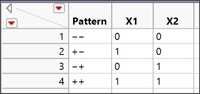The World Statistics Day celebration continues here in the Community. We all need reliable data for sound decision making. Do you have a data source that you trust most? Head over to Discussions to tell us about it.
Choose Language Hide Translation Bar
Highlighted

## Analyzing results when interaction is not possible

Hi!

A newbie question.

I have a basic experiment with 2 factors:The problem is that a combination of X1 and X2 technically is impossible.

Can I still analyze the results of first 3 patterns using regression? Or is there a differnt approach to this problem?

Actually this is so called ABN test on a site, Original (A), new feature version B(X1), version С(X2) the same new feature as B(X1) but is displayed to a user in a differnt way.

Learning DOE
1 ACCEPTED SOLUTION

Accepted Solutions
Highlighted

## Re: Analyzing results when interaction is not possible

The data determine what is estimable. Omitting one of the runs will limit the model that can be fit. It is OK, though. Remove the term that would cause the singularity. For example, if you omit either the (-1,-1) or the (+1,+1) run, then you must remove the interaction term from the model or else it will cause a singularity in the linear regression. So the nature of each run is important in defining which effect is estimable.

Remember that with only three runs, it is possible to estimate the intercept and the two main effects. It is impossible to perform any hypothesis tests on the model as a whole or on individual terms. So the number of runs is important in defining which tests are possible.

Learn it once, use it forever!
Highlighted

## Re: Analyzing results when interaction is not possible

The data determine what is estimable. Omitting one of the runs will limit the model that can be fit. It is OK, though. Remove the term that would cause the singularity. For example, if you omit either the (-1,-1) or the (+1,+1) run, then you must remove the interaction term from the model or else it will cause a singularity in the linear regression. So the nature of each run is important in defining which effect is estimable.

Remember that with only three runs, it is possible to estimate the intercept and the two main effects. It is impossible to perform any hypothesis tests on the model as a whole or on individual terms. So the number of runs is important in defining which tests are possible.

Learn it once, use it forever!
Article Labels

There are no labels assigned to this post.